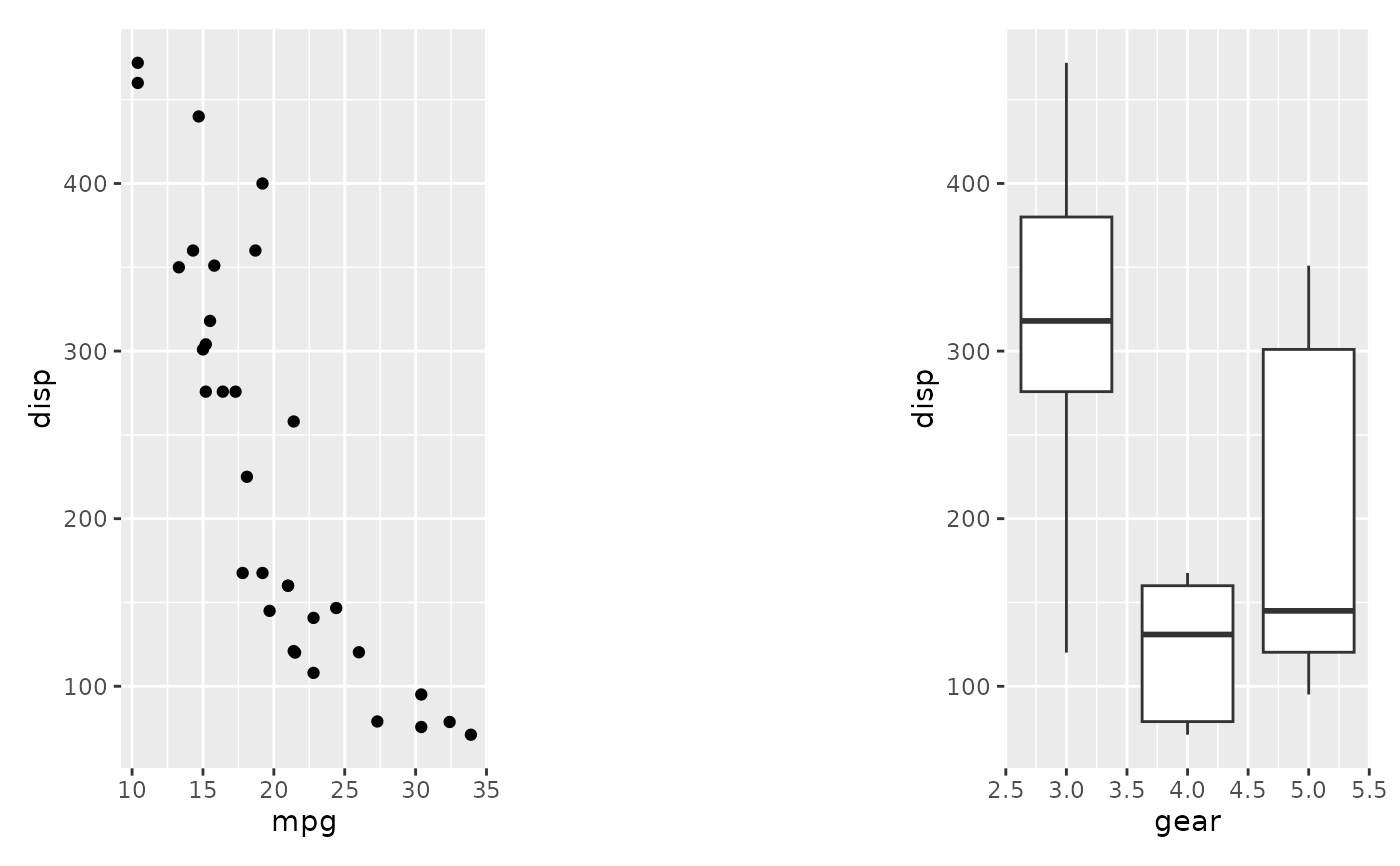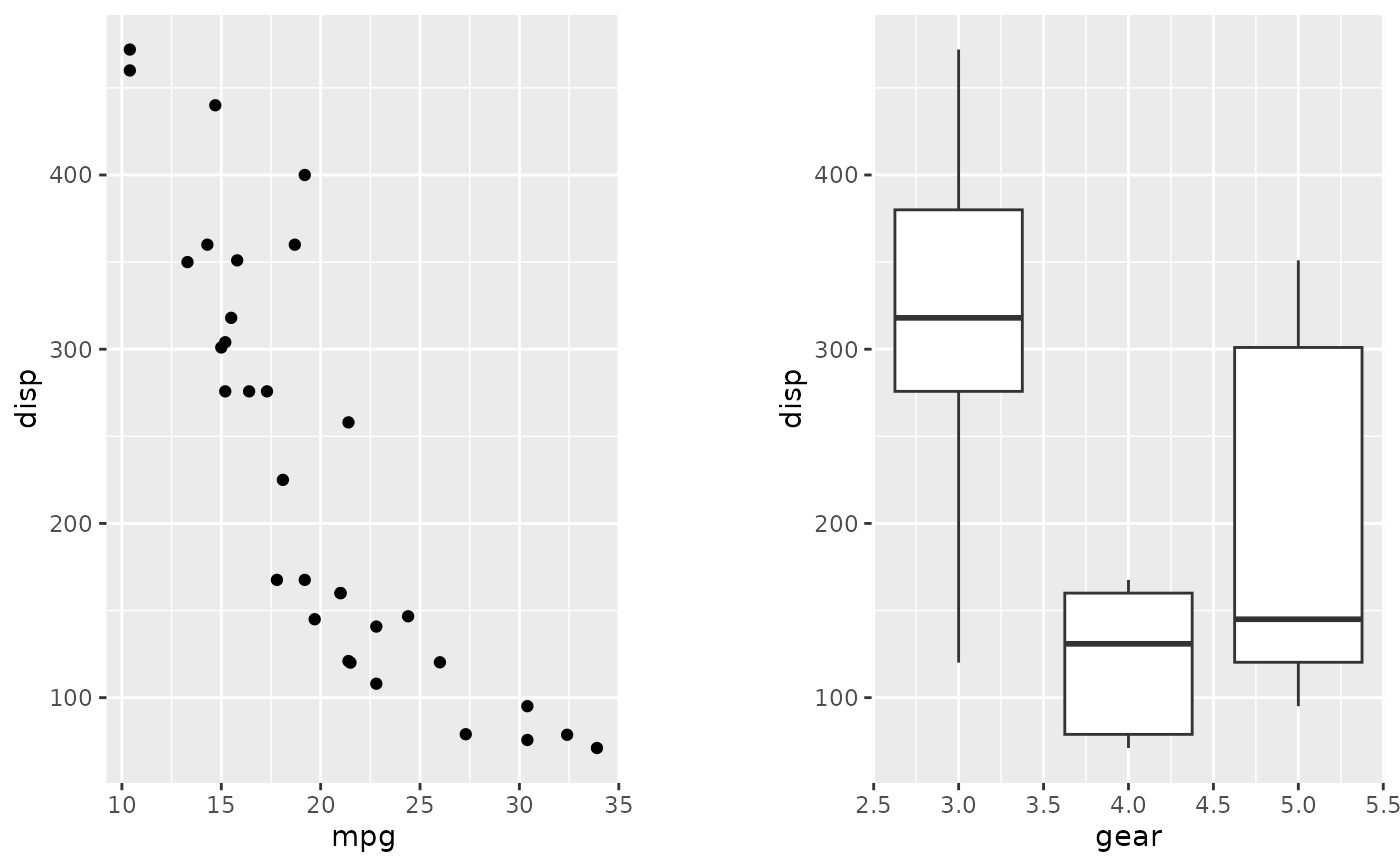This simple wrapper creates an empty transparant patch that can be added to push your other plots apart. The patch responds to adding theme() specifications, but only plot.background will have an effect.

plot_spacer()

## Value

A ggplot object containing an empty plot

## Examples

library(ggplot2)

p1 <- ggplot(mtcars) + geom_point(aes(mpg, disp))
p2 <- ggplot(mtcars) + geom_boxplot(aes(gear, disp, group = gear))

p1 + plot_spacer() + p2# To have more control over spacing, you can use the plot.margin
# parameter for theme() on each individual plot.

(p1 + theme(plot.margin = unit(c(0,30,0,0), "pt"))) +
(p2 + theme(plot.margin = unit(c(0,0,0,30), "pt")))